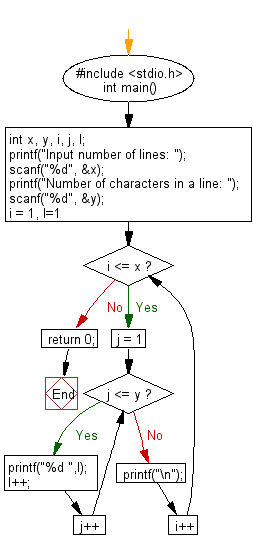﻿ C : Prints p numbers of lines 1 through q in a sequence

# C Exercises: Reads two integers p and q, print p number of lines in a sequence of 1 to q in a line

## C Basic Declarations and Expressions: Exercise-43 with Solution

Write a C program that reads two integers p and q, prints p number of lines in a sequence of 1 to b in a line.

Sample Solution:

C Code:

``````#include <stdio.h>
int main() {
int x, y, i, j, l;

// Prompt for user input
printf("Input number of lines: ");
scanf("%d", &x);
printf("Number of numbers in a line: ");
scanf("%d", &y);

// Loop to generate the pattern
for(i = 1, l=1; i <= x; i++) {
for(j = 1; j <= y; j++) {
printf("%d ", l);
l++;
}
printf("\n");
}

return 0;
}
``````

Sample Output:

```Input number of lines: 5
Number of numbers in a line: 6
1 2 3 4 5 6
7 8 9 10 11 12
13 14 15 16 17 18
19 20 21 22 23 24
25 26 27 28 29 30
```

Flowchart:C programming Code Editor:

What is the difficulty level of this exercise?

Test your Programming skills with w3resource's quiz.

﻿